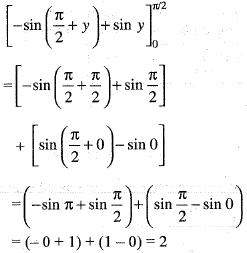Courses

# Test: Double And Triple Integrals - 1

## 20 Questions MCQ Test Topic-wise Tests & Solved Examples for IIT JAM Mathematics | Test: Double And Triple Integrals - 1

Description
This mock test of Test: Double And Triple Integrals - 1 for Mathematics helps you for every Mathematics entrance exam. This contains 20 Multiple Choice Questions for Mathematics Test: Double And Triple Integrals - 1 (mcq) to study with solutions a complete question bank. The solved questions answers in this Test: Double And Triple Integrals - 1 quiz give you a good mix of easy questions and tough questions. Mathematics students definitely take this Test: Double And Triple Integrals - 1 exercise for a better result in the exam. You can find other Test: Double And Triple Integrals - 1 extra questions, long questions & short questions for Mathematics on EduRev as well by searching above.
QUESTION: 1

Solution:
QUESTION: 2

Solution:
QUESTION: 3

### The area bounded by the curve y = ψ(x), x-axis and the lines x = l , x = m(l <m ) is given by

Solution:
QUESTION: 4

The volume of an object expressed in spherical coordinates is given by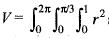sin φ dr dφ dθ. The value of the integral is

Solution:
QUESTION: 5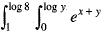dx dy is equal to

Solution:
QUESTION: 6

Using the transformation x + y = u, y = v. The value of Jacobian (J) for the integral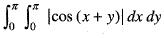is

Solution:
QUESTION: 7

The area bounded by the parabola y2 = 4ax and straight line x + y = 3a is

Solution:
QUESTION: 8

Consider the shaded triangular region P shown in the figure, what is the value of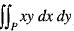?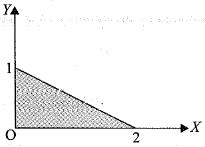Solution:
QUESTION: 9

Changing the order of the integration in the double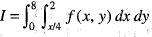leads to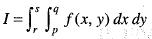. What is q?

Solution:

QUESTION: 10

To evaluate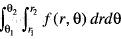over the region A bounded by the curve r = r1, r = r2 and the straight lines θ = θ1, θ = θ​2, we first integrate

Solution:
QUESTION: 11

To change Cartesian plane (x, y, z) to spherical polar coordinates (r, θ, φ)

Solution:

To convert a point from Cartesian coordinates to spherical coordinates, use equations

r2 = x2 + y2 + z2 , tanθ = y/x,   φ = arccos(z/√(x2+y2+z2) )

QUESTION: 12

The double integral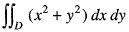,where D is bounded by y = x and y2 = 4x is equal to

Solution:
QUESTION: 13

What is the total mass of cube between the limits 0≤ x≤ 1, 0≤ y≤ 1, 0 ≤z ≤1 at any point given by xyz?

Solution:
QUESTION: 14

Area bounded by the curves y2 = x3 and x2 = y3 is

Solution:
QUESTION: 15

The value of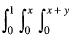(x+y+z) dzdydx is

Solution:
QUESTION: 16

By changing the order of integration in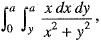the value is

Solution:
QUESTION: 17

By the change of variable x(u, v) = uv, y(u, v) = u/v is double integral, the integrand f(x, y) change to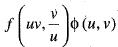. Then, φ(u,v) is

Solution:
QUESTION: 18

The volume of the tetrahedron bounded by the plane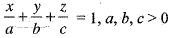and the co-ordinate planes is equal to

Solution:

Here
Let u = x/a, v = y/b , w = z/c
Then dx = a du, dy = b dv, dz = c dw
So, Required volume
V =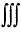abc du dv dw
where u + v + w ≤ 1, u ,v ,w ≥ 0
Thus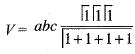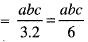Hence, the correct answer is (c)

QUESTION: 19

The value of integral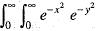dxdy is

Solution:

I =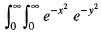dxdy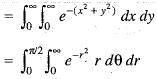Putting x+ y2 = r2, we get
Limits of  r = 0 to ∞
and 0 = θ to π/2
Now, Putting r2 = t, we get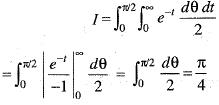QUESTION: 20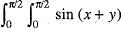dxdy is

Solution: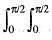sin (x+y) dxdy
Firstly integrating w.r.t. x, we get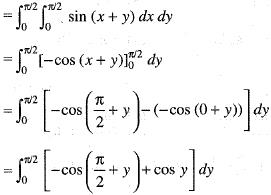Now , integrating w.r.t. y, we get,